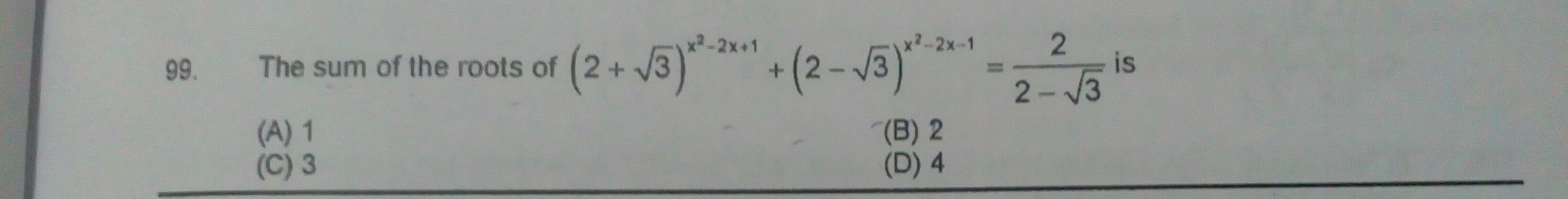# The sum of roots of 2+√3)x²-2x+1+(2-√3)x²-2x-1=2/2-√3 is4 years ago

〖(2+√3)〗^(x^2-2x+1)+〖(2-√3)〗^(x^2-2x-1)=2/(2-√3)

The above equation can be rewritten as

〖(2+√3)〗^(x^2-2x)*(2+√3)+〖(2-√3)〗^(x^2-2x)*(2-√3)-1 = 2/(2-√3)

Multiply the equation with 2-√3

We get〖(2+√3)〗^(x^2-2x)*(2+√3)(2-√3)+〖(2-√3)〗^(x^2-2x)*(2-√3)-1(2-√3)=4

Or 〖(2+√3)〗^(x^2-2x)*(4-3)+ 〖(2-√3)〗^(x^2-2x)*(2-√3)-1+1=4 ( Using (a+b)*(a-b)=a^2-b^2 in the first part on left side and law of indices in second part a^m*a^n=a^(m+n) and remembering that a^0=1)

〖(2+√3)〗^(x^2-2x)+〖(2-√3)〗^(x^2-2x)=2

In second part on left side we can multiply numerator and denominator with conjugate 2+√3

The equation now becomes

〖(2+√3)〗^(x^2-2x)+〖[(2-√3)(2+√3)/(2+√(3)])〗^(x^2-2x)=2

We again use (a+b)*(a-b)=a^2-b^2 in the second part on LHS (2-√3)(2+√3)=4-3=1

The equation now can be re-written as

〖(2+√3)〗^(x^2-2x)+〖[1/(2+√(3)])〗^(x^2-2x)=2

Let (2+√3)^(x^2-2x)=y

The above equation is now

Y+1/y = 2

Or (y²+1)/y =2

Arranging the above equation we get

Y²-2Y +1 = 0

hope you can do iy now